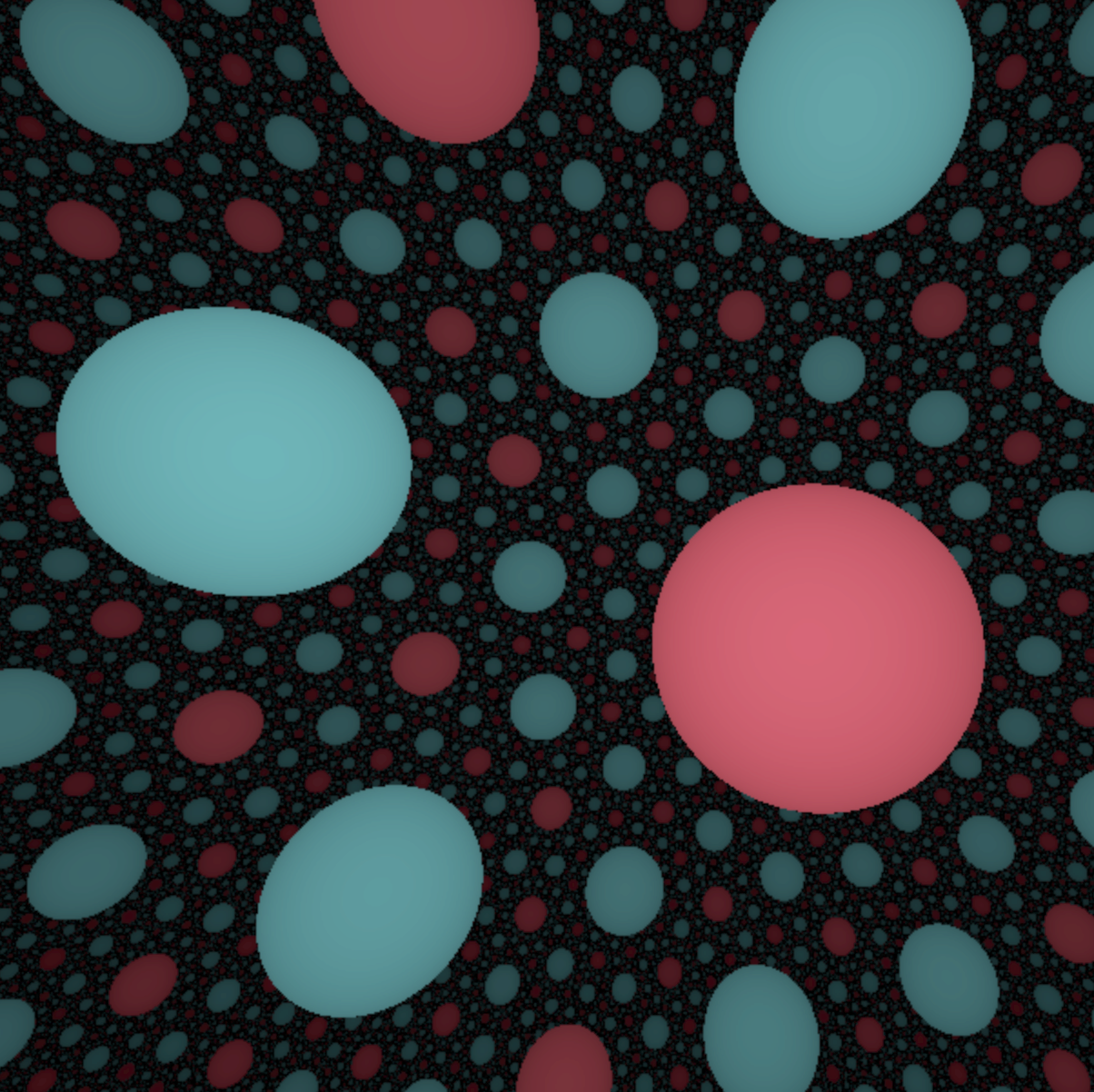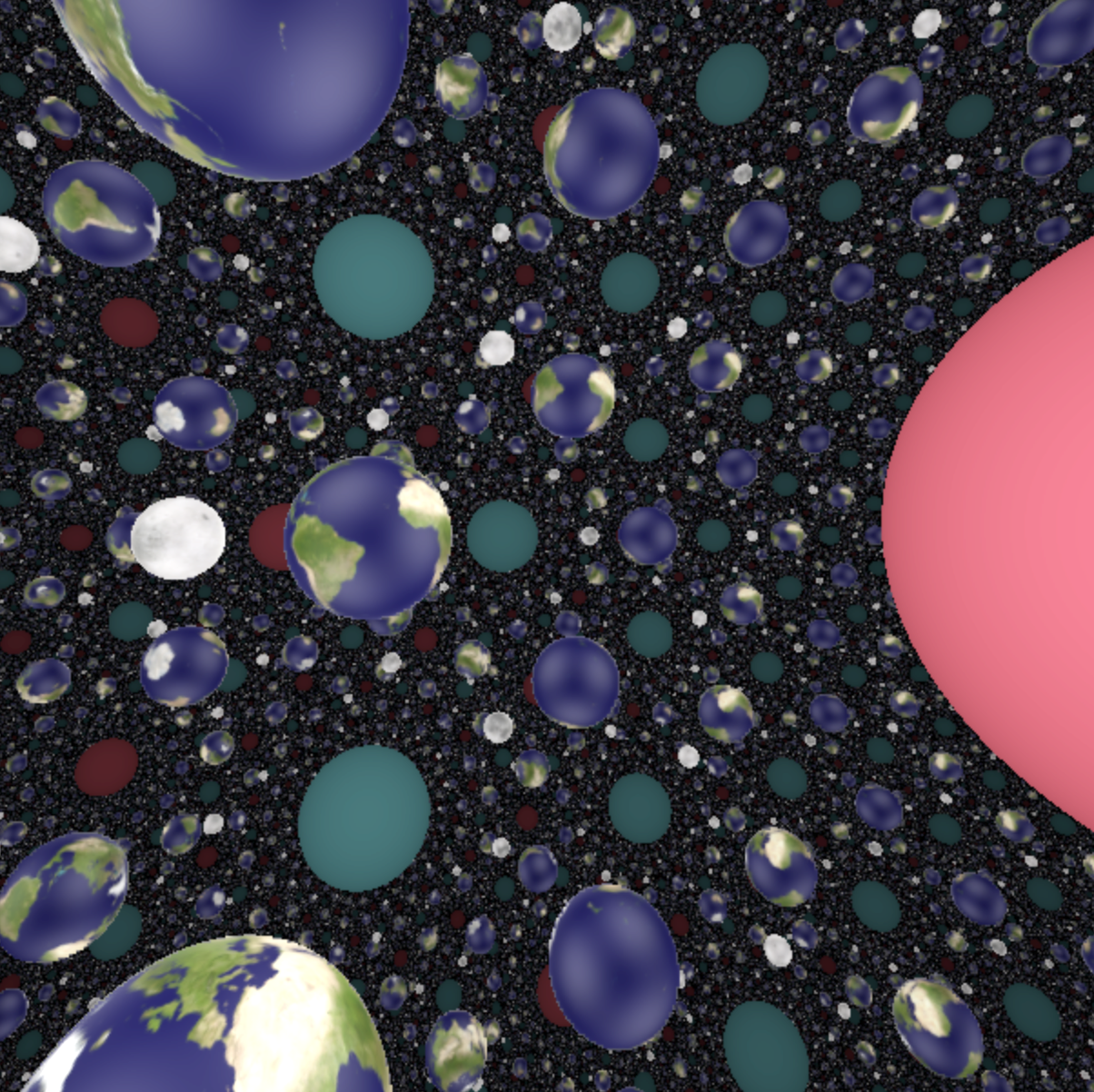# The hyperbolic space $\mathbb H^3$

## What is $\mathbb H^3$?

The hyperbolic space $\mathbb H^3$ is the three-dimensional analog of the hyperbolic plane. It is an isotropic space (all the directions play the same role). Among the eight geometries, this is probably the one which has the richest class of lattices.

Click on the button below to reveal a concrete model of the hyperbolic space.

There are multiple models for the hyperbolic space: the Poincaré ball model, the upper half-space model, the projective model, etc. We chose to work with the hyperboloid model. In this model, $\mathbb H^3$ corresponds to one sheet of a hyperboloid, given by the following equations. $$X = \left\{ (x,y,z,w) \in \mathbb R^4 \mid x^2 + y^2 + z^2 - w^2 = - 1, \ w > 0 \right\}.$$ The lorentzian metric on $\mathbb R^4$ given by $$ds^2 = dx^2 + dy^2 + dz^2 - dw^2.$$ induces a riemanian metric on $X$ Its isometry group is $SO(3,1)$, i.e. the group of linear transformations of $\mathbb R^4$ preserving the lorentzian form. This group acts transitively on the unit tangent bundle of $X$. Thus $X$ is not only homogeneous but also isotropic.

## Some views of $\mathbb H^3$

Warning: Some of the real-time simulations below requires a powerful graphic card. If your computer is not fast enough, you can reduce the size of your browser window. Click on the button below to reveal the fly commands.

The default controls to fly in the scene are the following. You can choose your keyboard in the Option controls pannel in the top right corner of the window

Command QWERTY keyboard AZERTY keyboard
Yaw left a q
Yaw right d d
Pitch up w z
Pitch down s s
Roll left q a
Roll right e e
Move forward arrow up arrow up
Move backward arrow down arrow down
Move to the left arrow left arrow left
Move the the right arrow right arrow right
Move upwards ' ù
Move downwards / =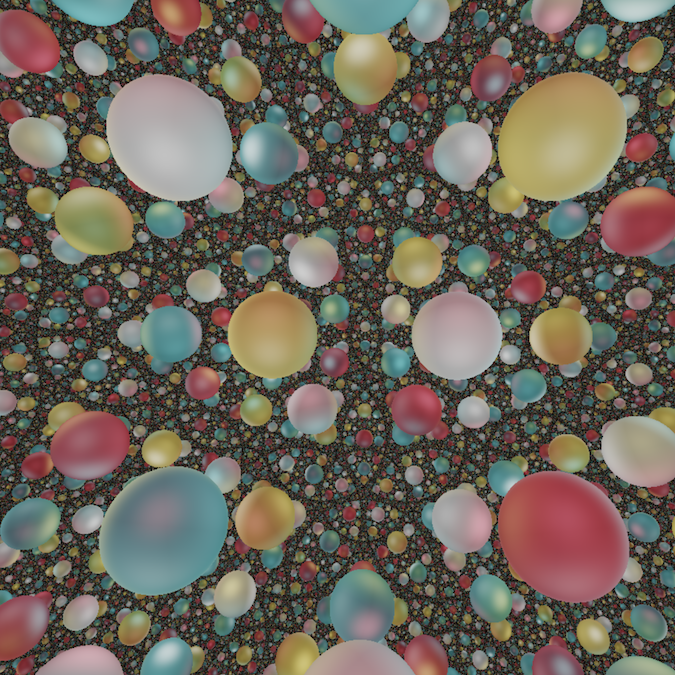##### Spheres

Twelve Spheres in Seifert Weber Dodecahedral Space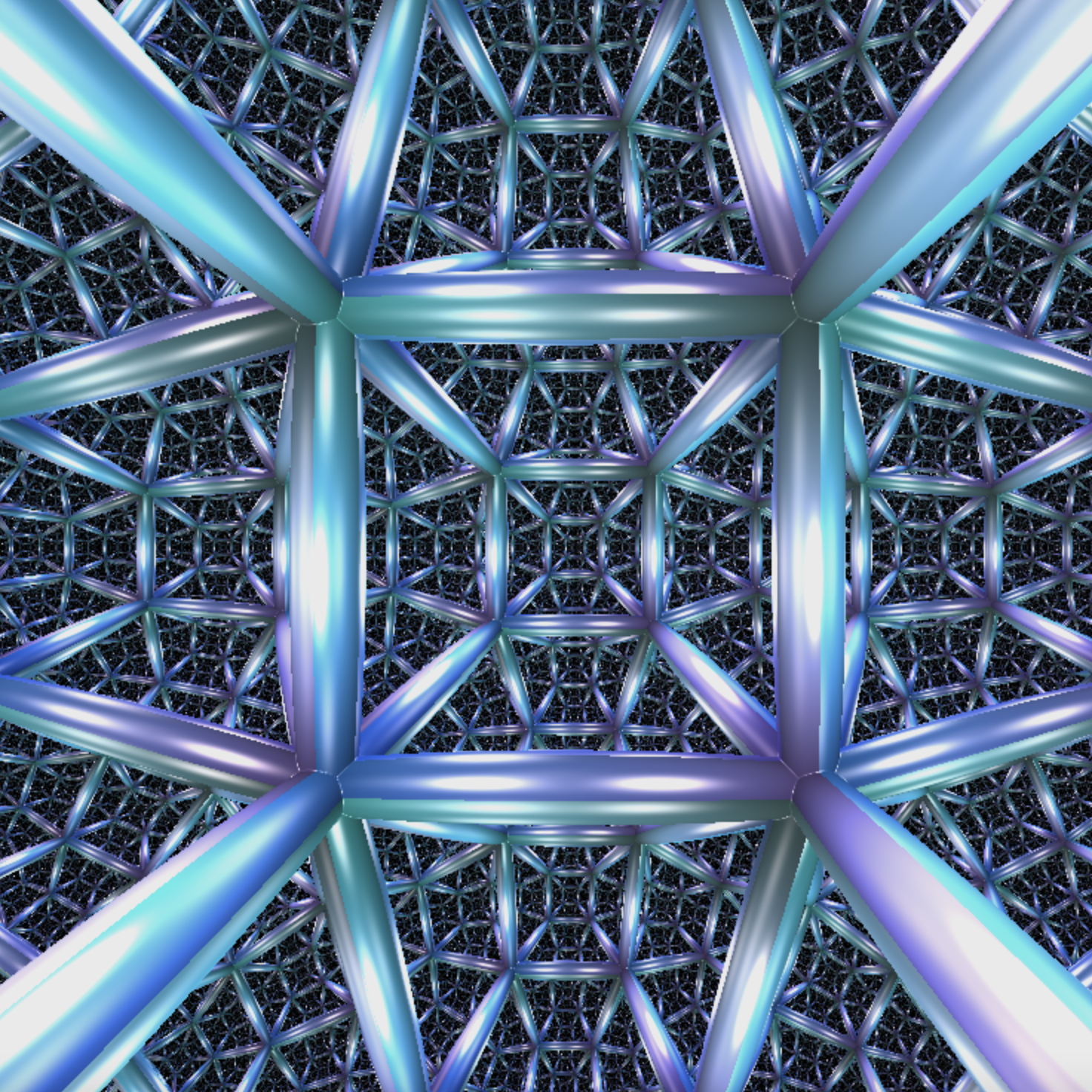##### Coxeter Tiling by Cubes

Finite cubes, no cusps

HD pictures of $\mathbb H^3$ can be found in the gallery

## Features of $\mathbb H^3$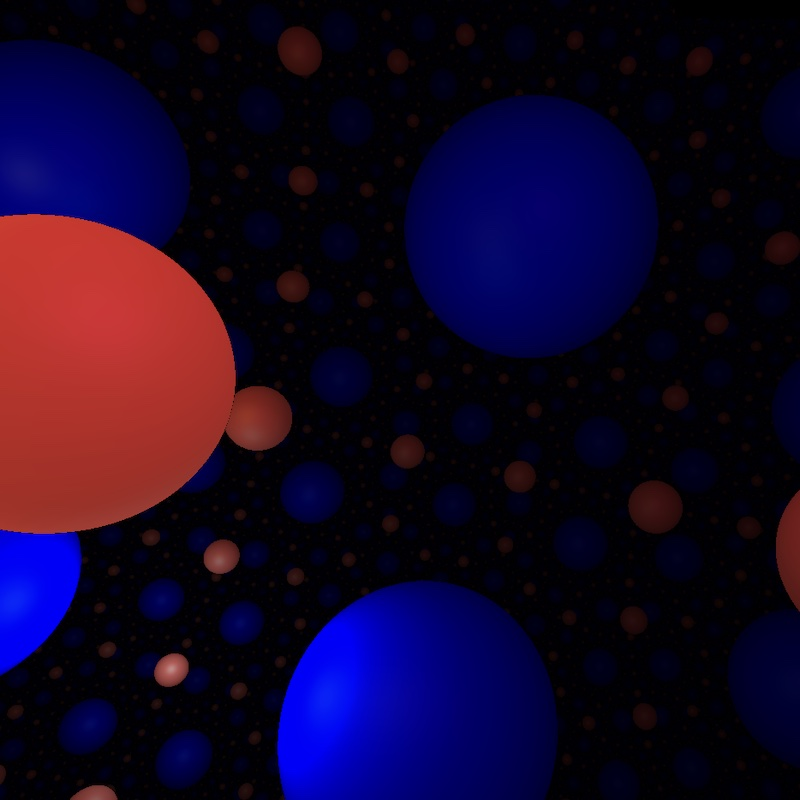##### Horospheres in $\mathbb{H}^3$

Non-Geodesic Euclidean Planes##### Lighting in $\mathbb{H}^3$

Effects of Exponential Divergence

Some features of $\mathbb H^3$ are described in the following Bridges paper by two members of our team (Henry and Sabetta) together with Vi Hart and Andrea Hawksley.

### Cusps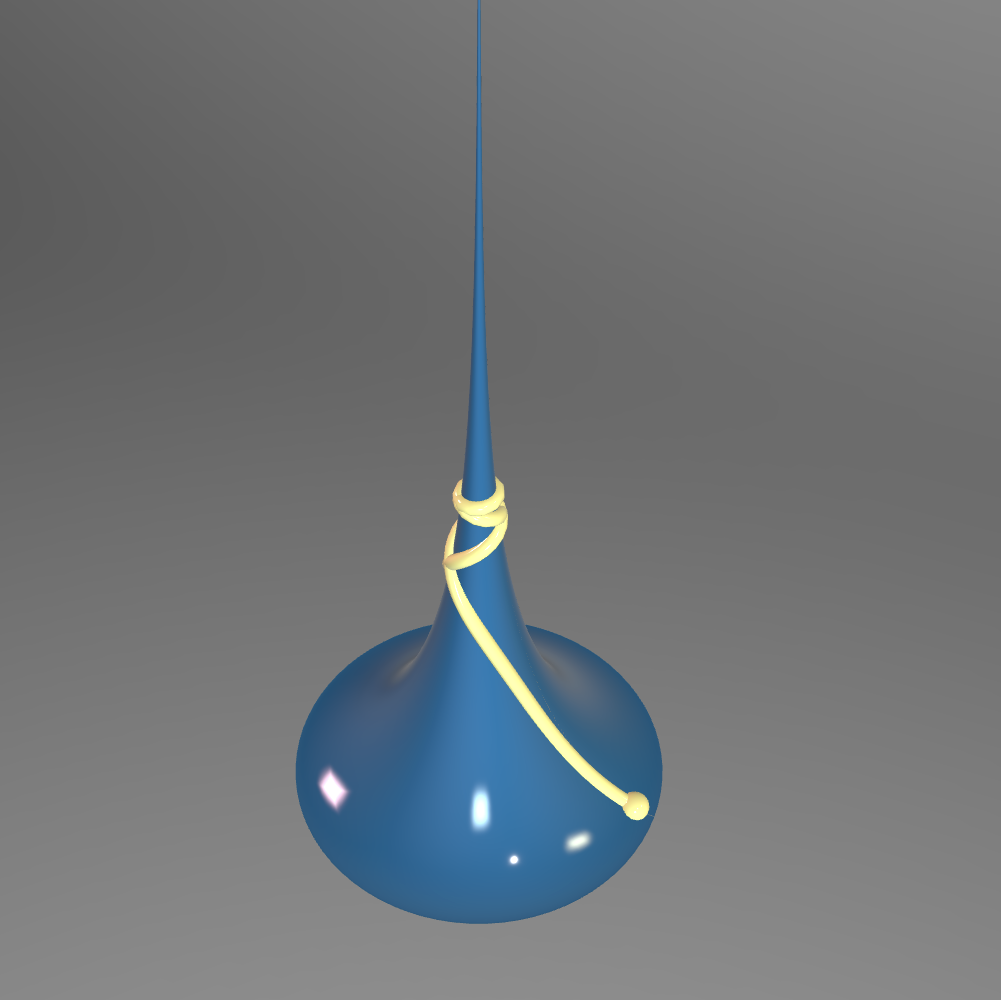##### A 2d Cusp

The Pseudosphere in $\mathbb{R}^3$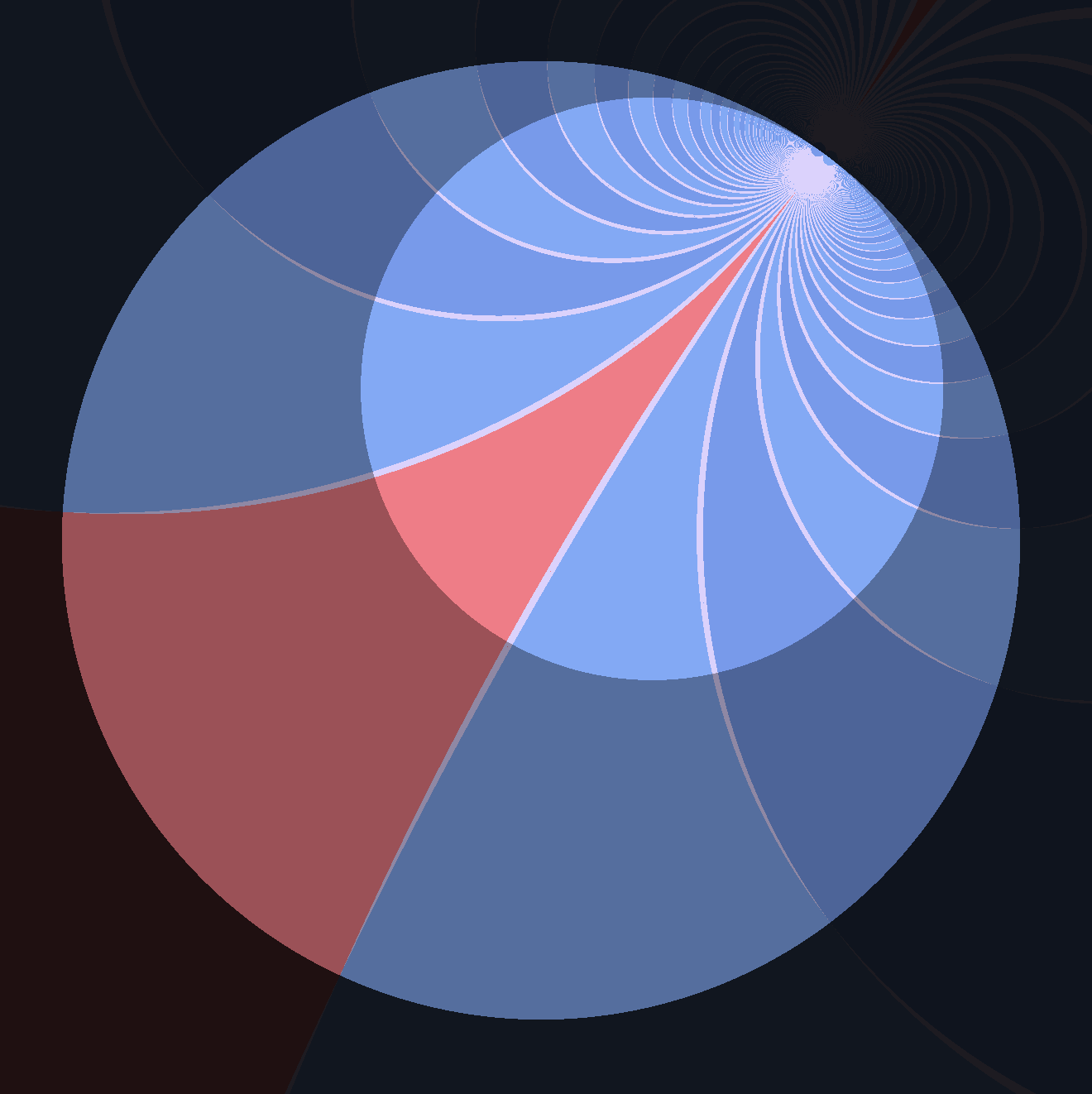##### A 2d Cusp

A Quotient of a Horoball\$

### Tilings and Polytopes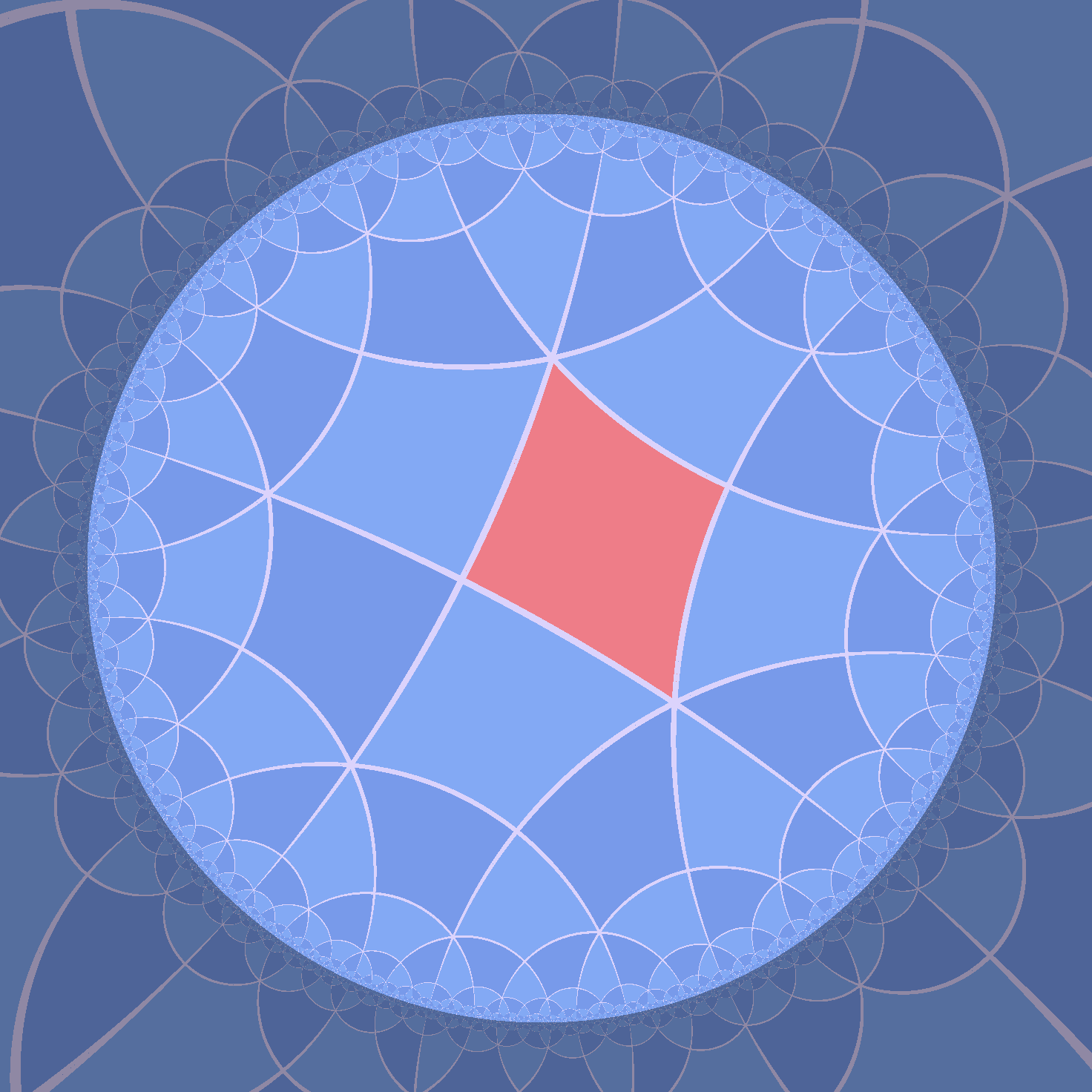A 2-dimensional example##### A Tiling by Cubes

RA Coxeter Groups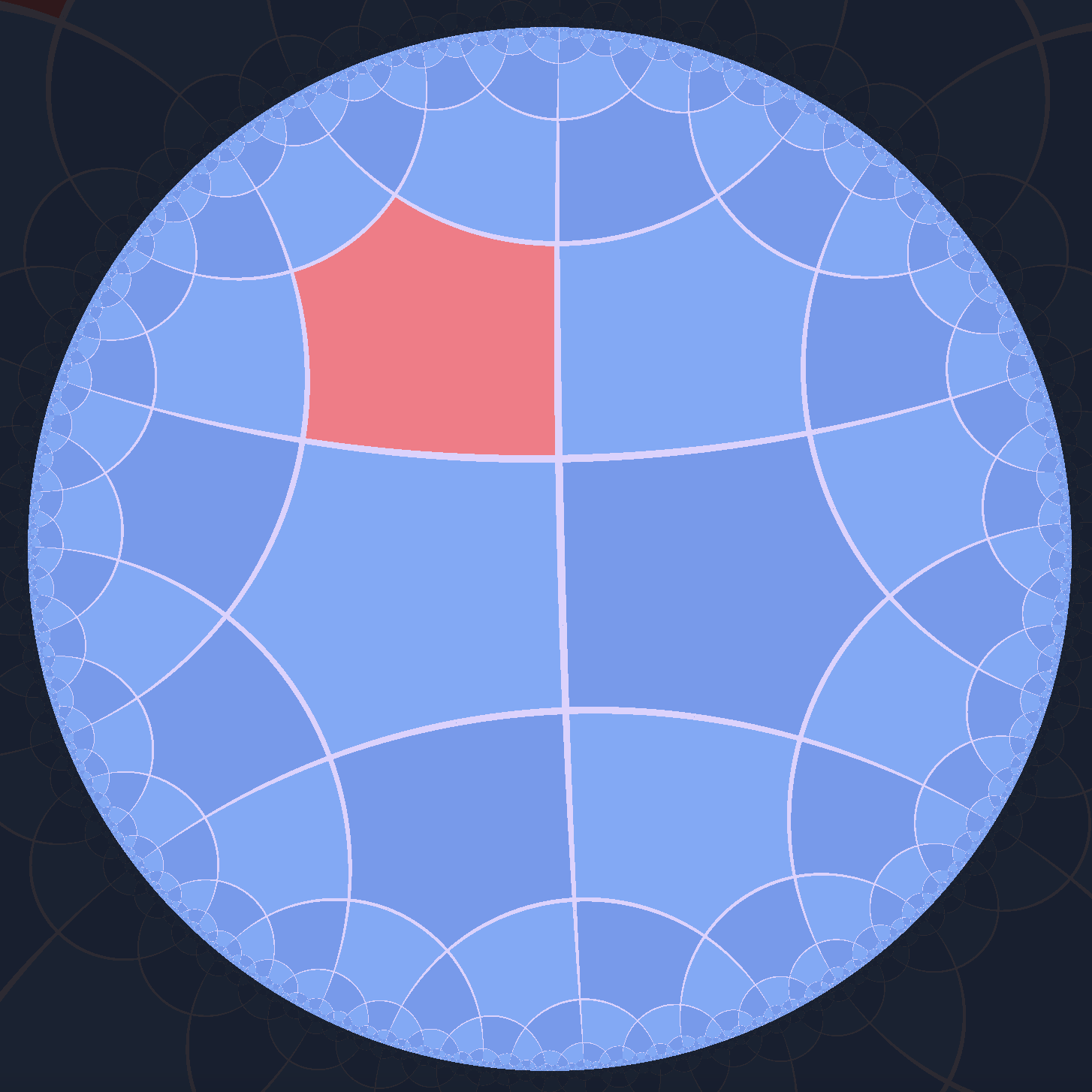##### Right Angled Pentagons

A 2-dimensional example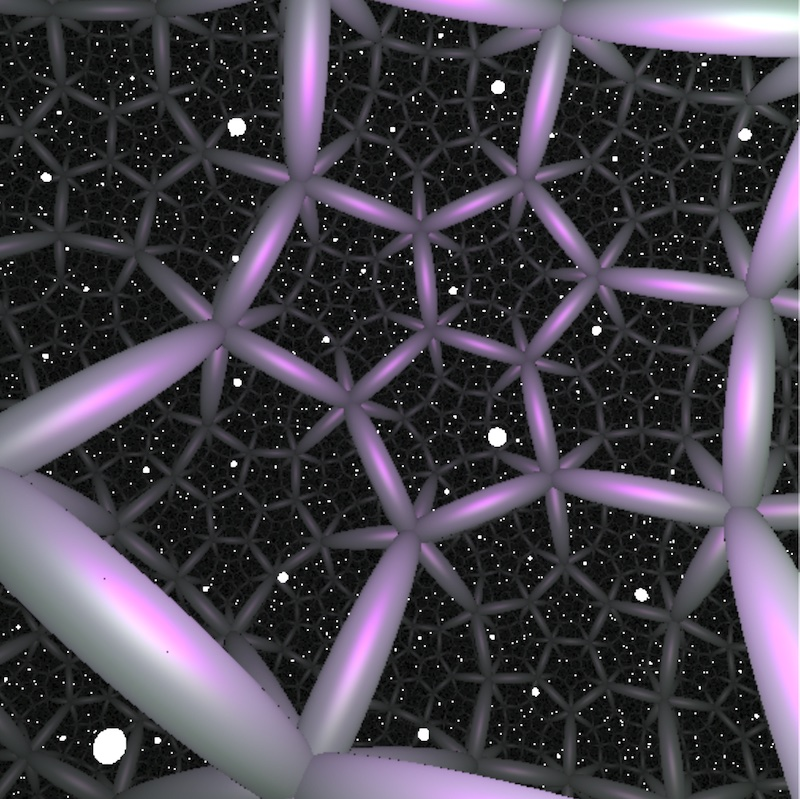##### A Tiling by Dodecahedra

Have a two-way view of the same fixed hyperbolic manifold (easiest, given what I have to do Whitehead Link complement): one: give an extrinsic view, of link complement and some other reference objects in the scene in S3. Two: an intrinsic view of the same thing, with the hyperbolic metric.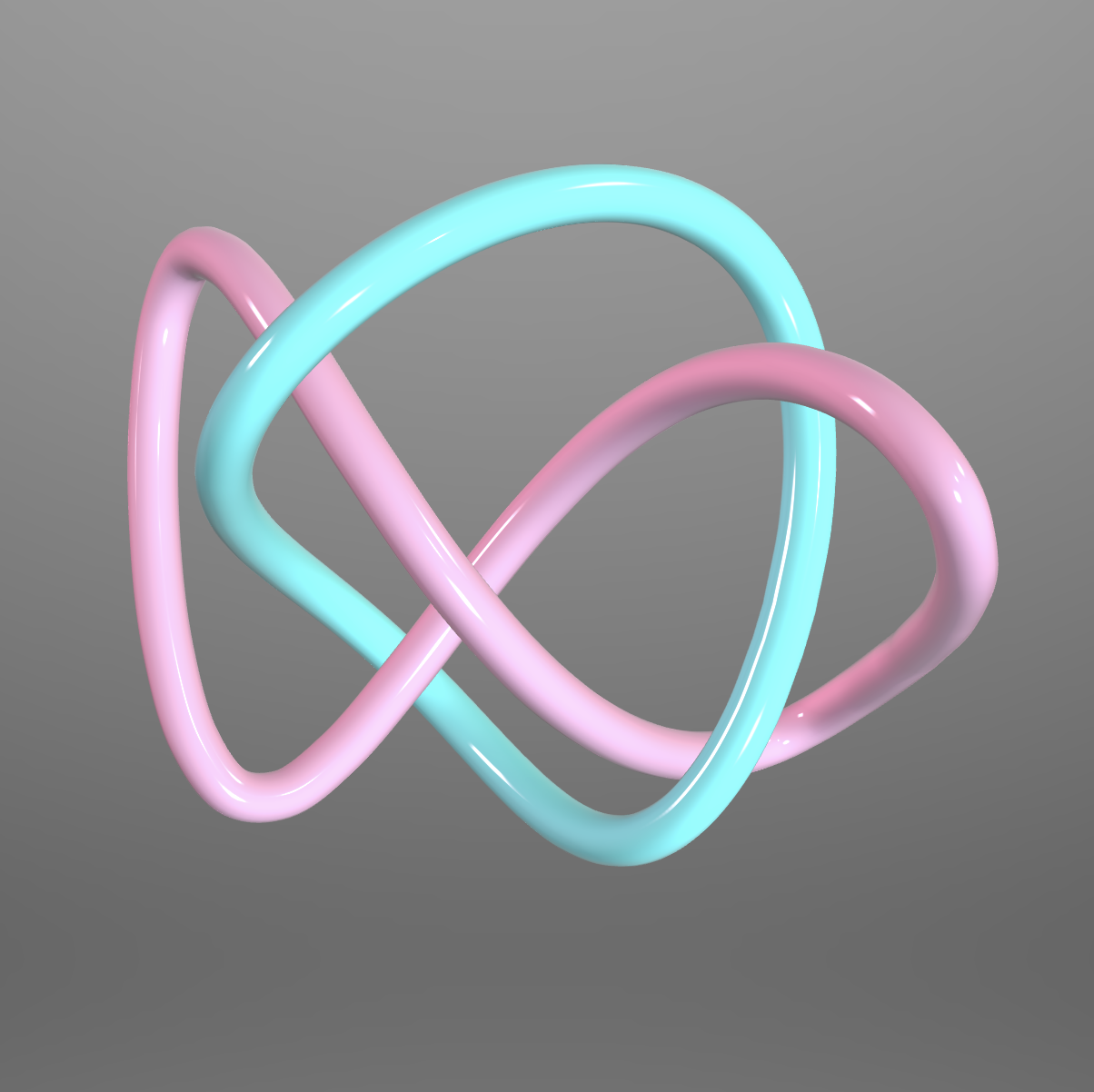##### Topological View